C Program to Count Positive Negative Zero

In this tutorial you will learn about the C Program to Count Positive Negative Zero and its application with practical example.

C Program to Count Positive Negative Zero

In this tutorial, we will learn to create a C program that will Count Positive Negative Zero using C programming.

Prerequisites

Before starting with this tutorial we assume that you are best aware of the following C programming topics:

• Operators in C Programming.
• Basic Input and Output function in C Programming.
• Basic C programming.
• Arithmetic operations in C Programming.
• While Loop in C programming.
• Conditional Statements in C programming.

Program to Count Positive Negative Zero

In c programming, it is possible to take numerical input from the user and Count Positive Negative Zero from the given number with the help of a very small amount of code. The C language has many types of header libraries which has supported function in them. With the help of these functions, the programming is easy.

With the help of this program, we can Count Positive Negative Zero.

Here,

We will first take the input

The second will make Count Positive Negative Zero.

Output:-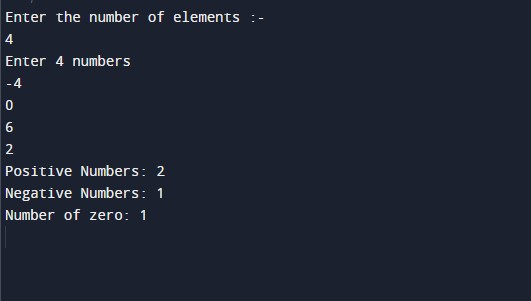In the above program, we have first initialized the required variable.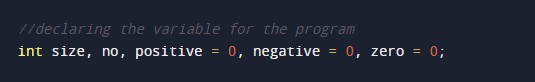• size = it will hold the integer value.
• no = it will hold the integer value.
• positive = it will hold the integer value.
• negative = it will hold the integer value.
• zero = it will hold the integer value.

Input number from the user.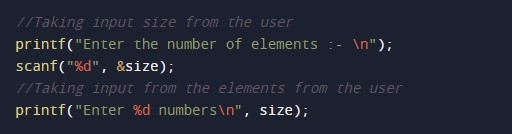Program Logic Code.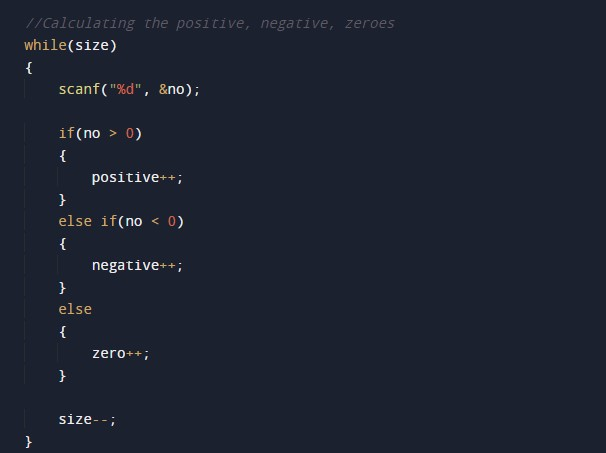Printing the output.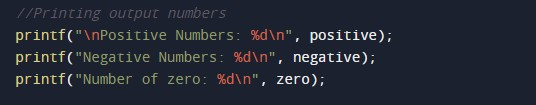In this tutorial we have learn about the C Program to Count Positive Negative Zero and its application with practical example. I hope you will like this tutorial.